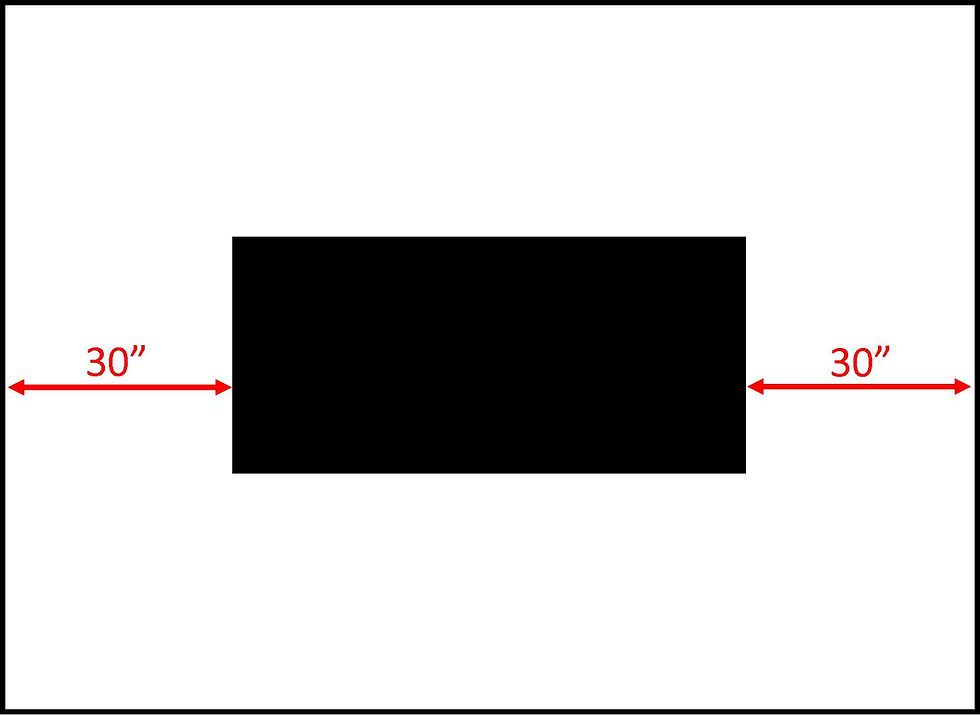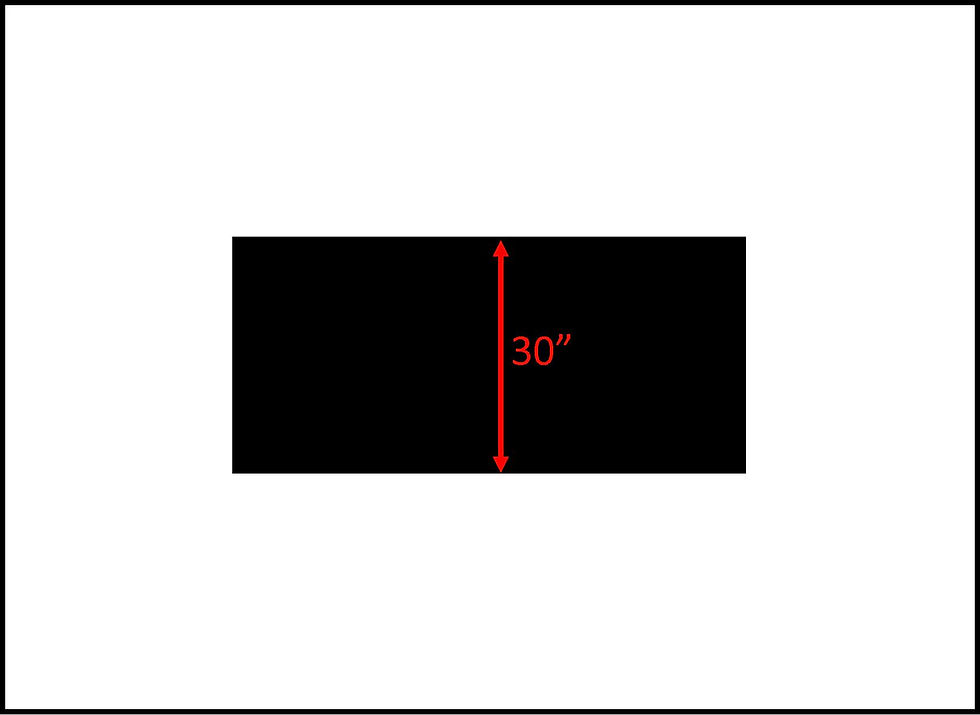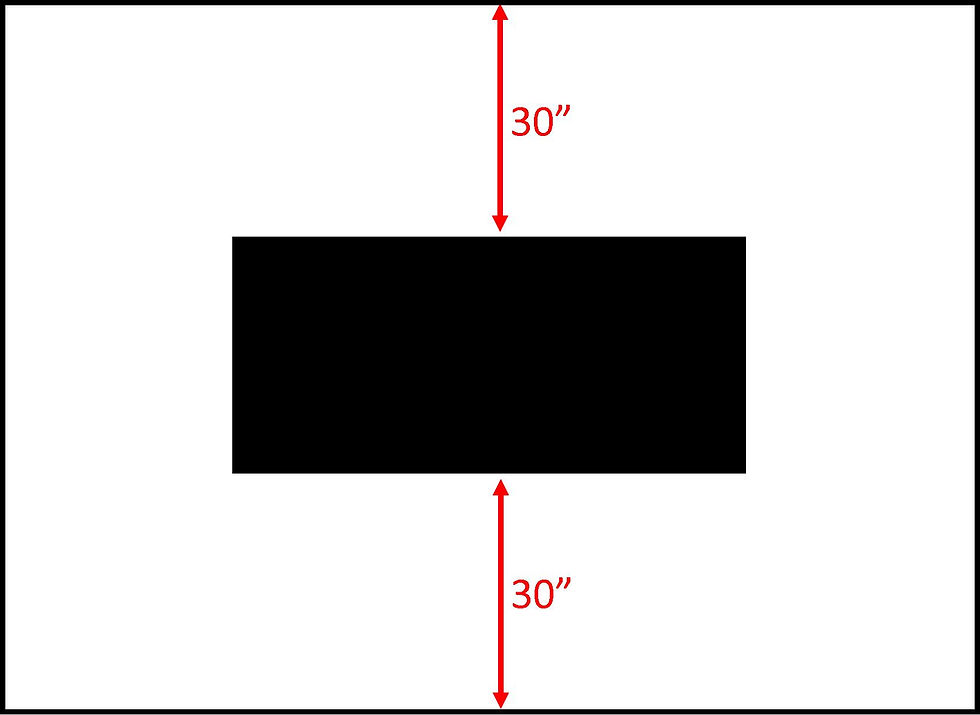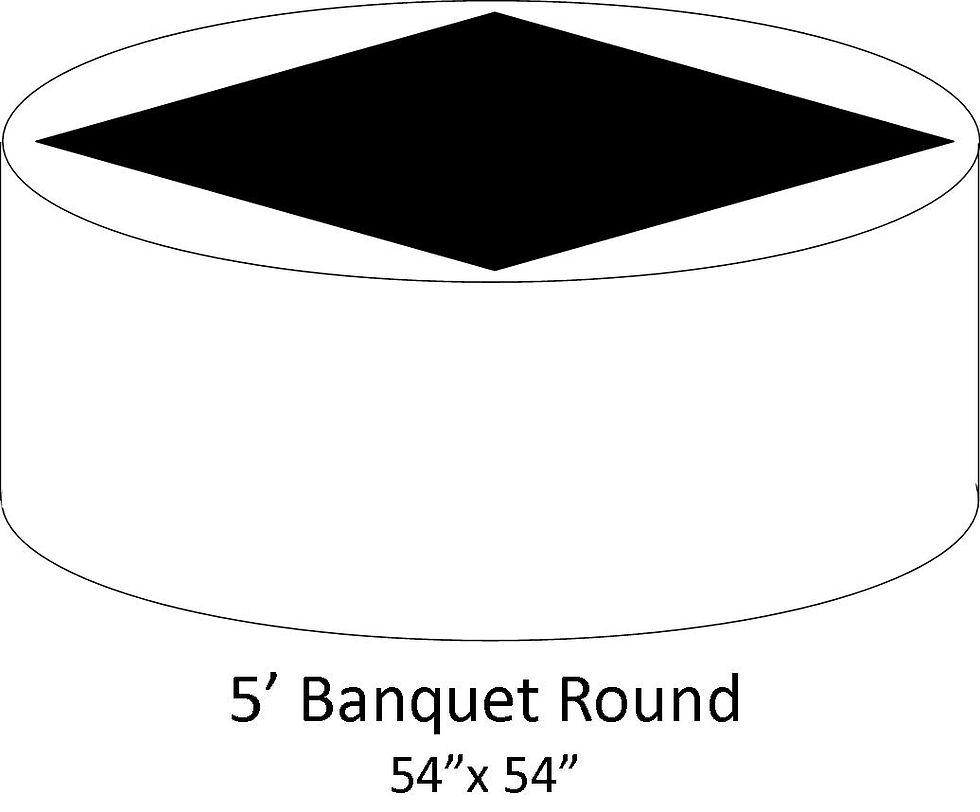top of page

# HOW TO: Choose the right linen size

Are linen shapes & sizes driving you insane? We don’t blame you. Sometimes WE even get confused, and linens are a major part of our job! That's why we want to help you, who doesn't look at this every day, in finding an easy solution to measure for the proper linen fit. We'll run through this exercise for rectangle/square tables, and also round. And at the very bottom of our blog, we'll show you how those different shapes lay on the tables.

### How to measure a rectangular table for floor-length linensWe'll start with a rectangular table (as seen here) and you can scroll down for a round table. This is how you calculate the size of a floor-length linen.To calculate measurements on your own, you need to convert everything to inches. We’ll do a few table sizes here:

8 feet = 96 inches

6 feet = 72 inches

5 feet = 60 inchesYou need to add the height on both sides of the table. Most banquet tables are 30” tall. So you’re usually adding 60” to your measurements (unless you don’t want a linen to be floor-length).Now we're able to calculate the LENGTH:

96 inches + 30 inches + 30 inches = 156” long

You calculate the length for this rectangular table, but now you need to calculate the width.Most banquet (rectangular tables) are 30” wide.Again, you need to add the height on both sides of the table.Now we're able to calculate the WIDTH:

30 inches + 30 inches + 30 inches = 90” wide

This means, a floor-length linen for an 8’ banquet table

is 90” x 156”

### How to measure a round table for floor-length linensThis is how you calculate the size of a floor-length linen for a round table.Measure the diameter of the table.Now add the height of the table on both sides.Now we're able to calculate the Floor-length linen size:

60 inches + 30 inches + 30 inches = 120”

This means, a floor-length linen for an 5’ round table 120”

1. Square linens come in sizes like 90”x 90” and 54”x 54.” Both numbers are the same (hence the square), but there are two numbers.

2. Round Linens have one measurement (diameter). So you’ll see numbers like 90” or 120”

3. Rectangular linens come in sizes like 90”x 132.” Obviously the shorter measurement for the width of the table and the longer measurement for the length.

4. Table runners typically come in 8” or 15” widths, and a length of 9 feet.

### So now that I know how to measure, what does it look like on the table?

Look at our handy dandy pictures below to find that perfect linen for the style you’re trying to achieve! We’re not going to go through ALL sizes, but we’ll go through the most popular options.